# 使用的设计流程：

deckBuild  -> command file  ->device simulator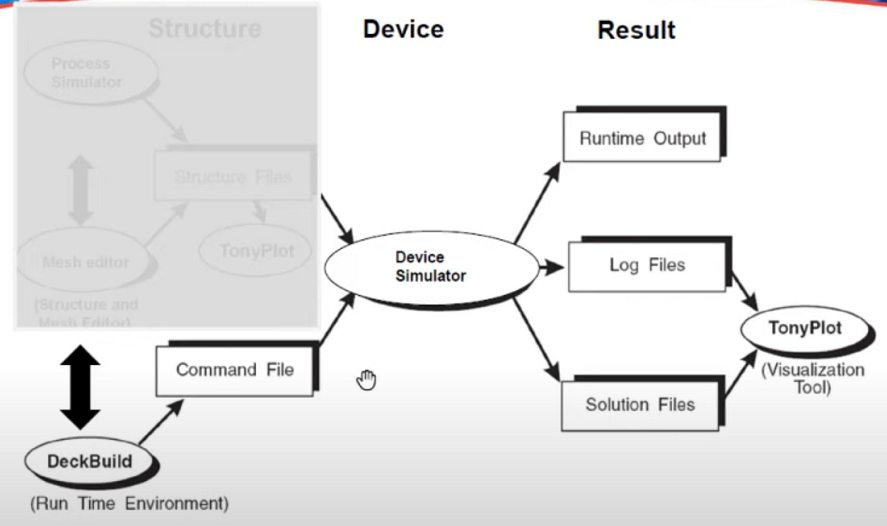# 在deckbuild 中运行atlas

1、打开deckbuild

Linux版本：

``terminal> deckbuild&``

windows版本：（我用的是windows版本）

2、运行atlas：

``go atlas``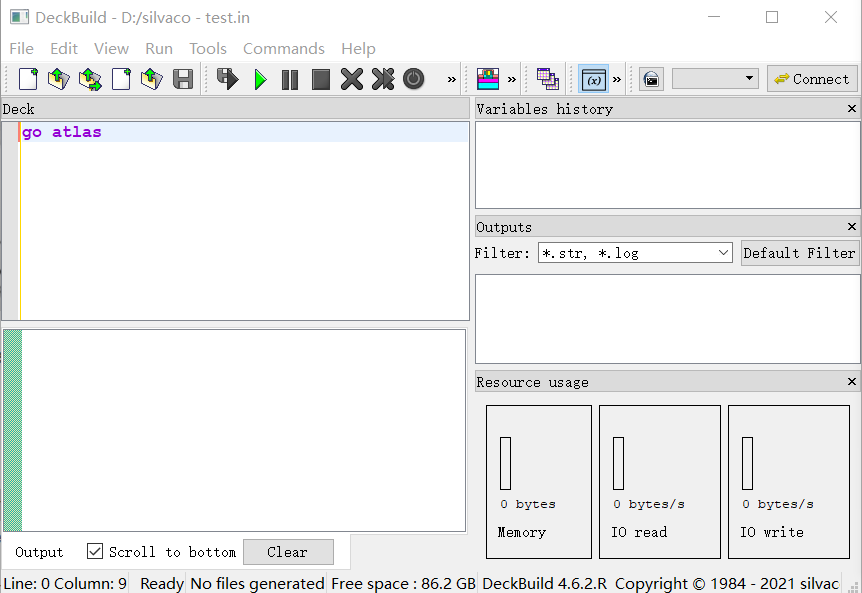设置CPU核数

``````go atlas simflags="-P 2"
//2个CPU
//默认情况下，是"P 1"``````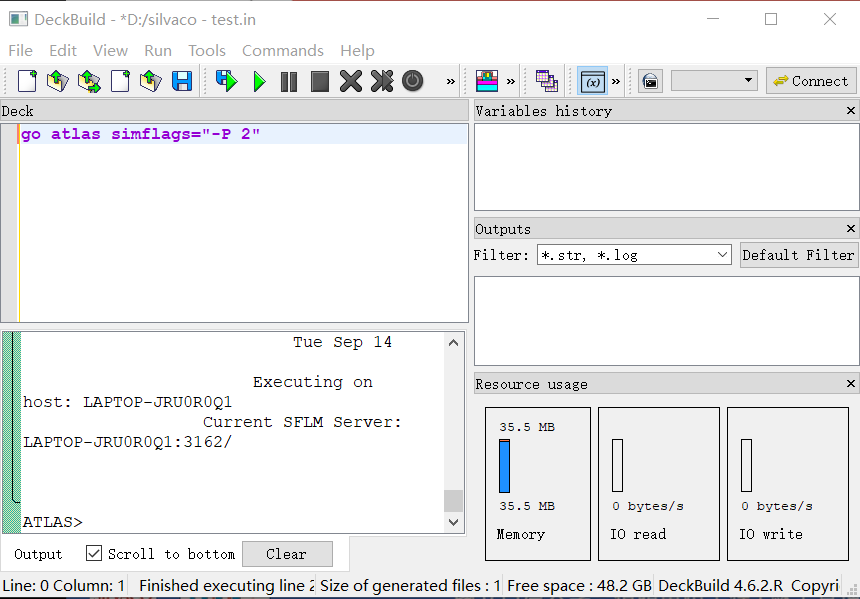# Atlas Design of Deck - Typical Flow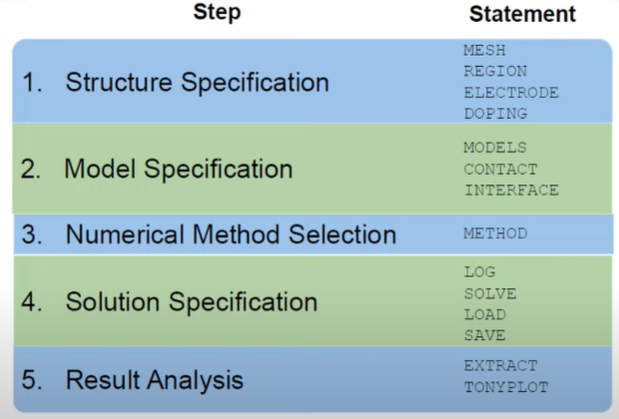## example nMOS

### 设计目标：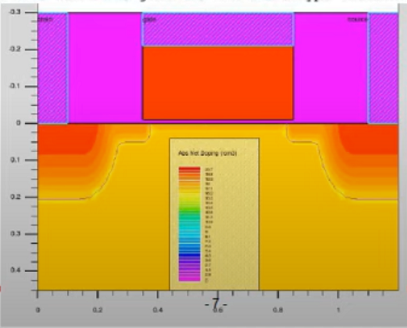### Structure Specification——mesh

• mesh
• region
• electrode
• doping

先把结构的大小定义出来，即layout design设计gate 是多少，source和drain与gate的间距是多少。

在仿真工艺之前需要先建立网格，这时的网格是指衬底的网格（如果后续工艺带来了新的材料层，则有相应的网格控制的参数）。

``````mesh width=l

x.mesh loc=0           spac=0.05  //x开始是0（也可以自己设定其他值）
x.mesh loc=0.1         spac=0.02
x.mesh loc=0.35        spac=0.01
x.mesh loc=0.6         spac=0.1
x.mesh loc=o.85        spac=0.01
x.mesh loc=l.l         spac=0.02
x.mesh loc=l.2         spac=0.1  //x结束是1.2

//定义y
y.mesh loc=-0.3        spac=0.05
y.mesh loc=-0.21       spac=0.05
y.mesh loc=-0.01       spac=0.002
y.mesh loc=0           spac=0.002
y.mesh loc=10          spac=l

/*
loc：设定网格线在轴上的坐标，看成比较大的区间

spac： 设定在该 loc 处临近网格线间的间距。可以看成比较小的区间（对比较大的区间的再划分）

loc 和 spacing 的默认单位都是 μm

每个材料的切分点都要有一个mesh，按照X轴和Y轴进行划分的（x向右为正，y向下为正）

整个器件，在X轴上为0到1.2um，
分别放了几条线用于确定相关的结构，比如栅极和源极的范围
将这几个区间进行了不同程度的细化，如0到0.1，对其每0.05um划分一次，然后Y轴也同理，
注意的Y轴的0处，因为Y轴只有0坐标处可以进行高斯分布的掺杂，因此建议在0处以上就是一些不需要掺杂或者可以直接进行均匀掺杂的部分。
*/``````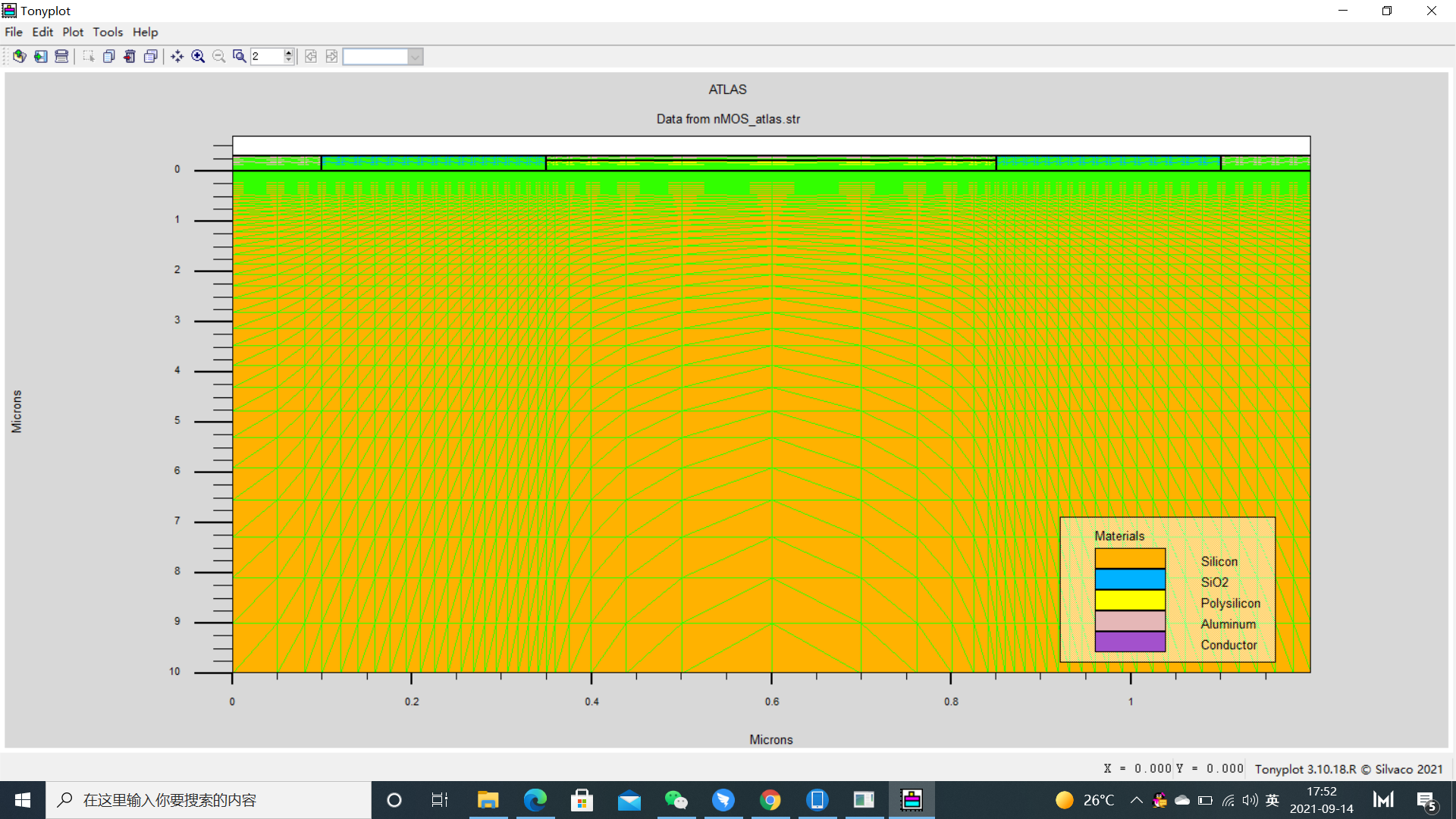网格切的越细，计算越精确，可让doping profile那些越接近现实。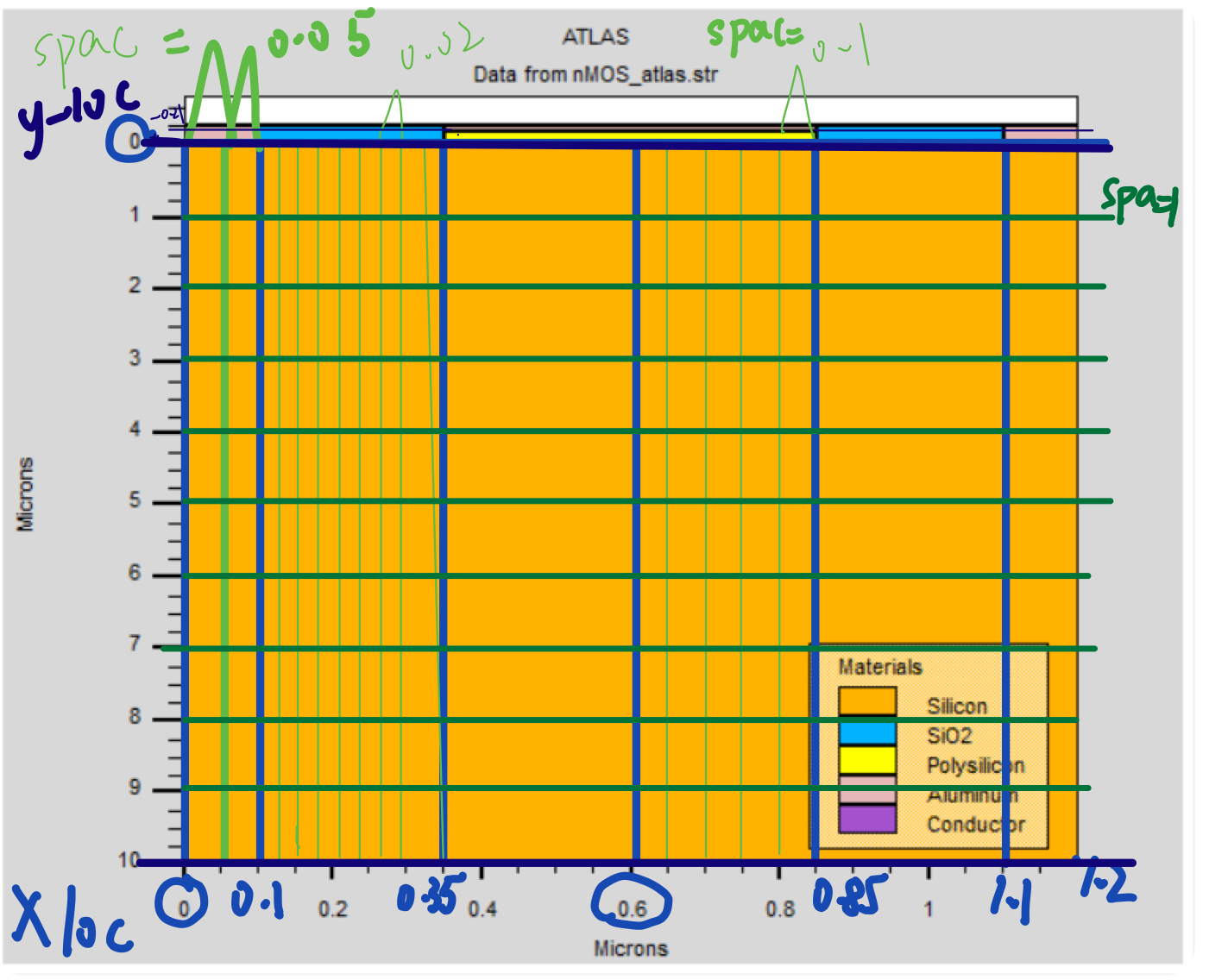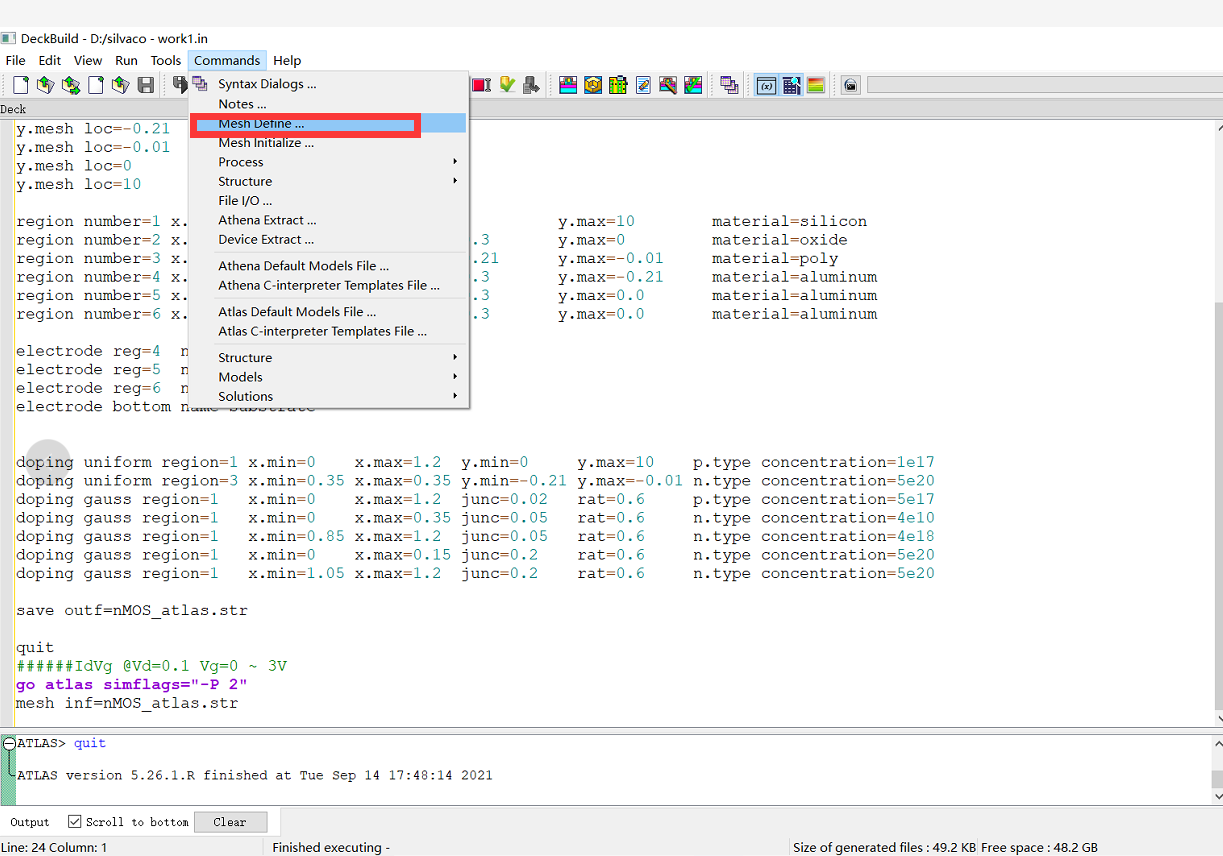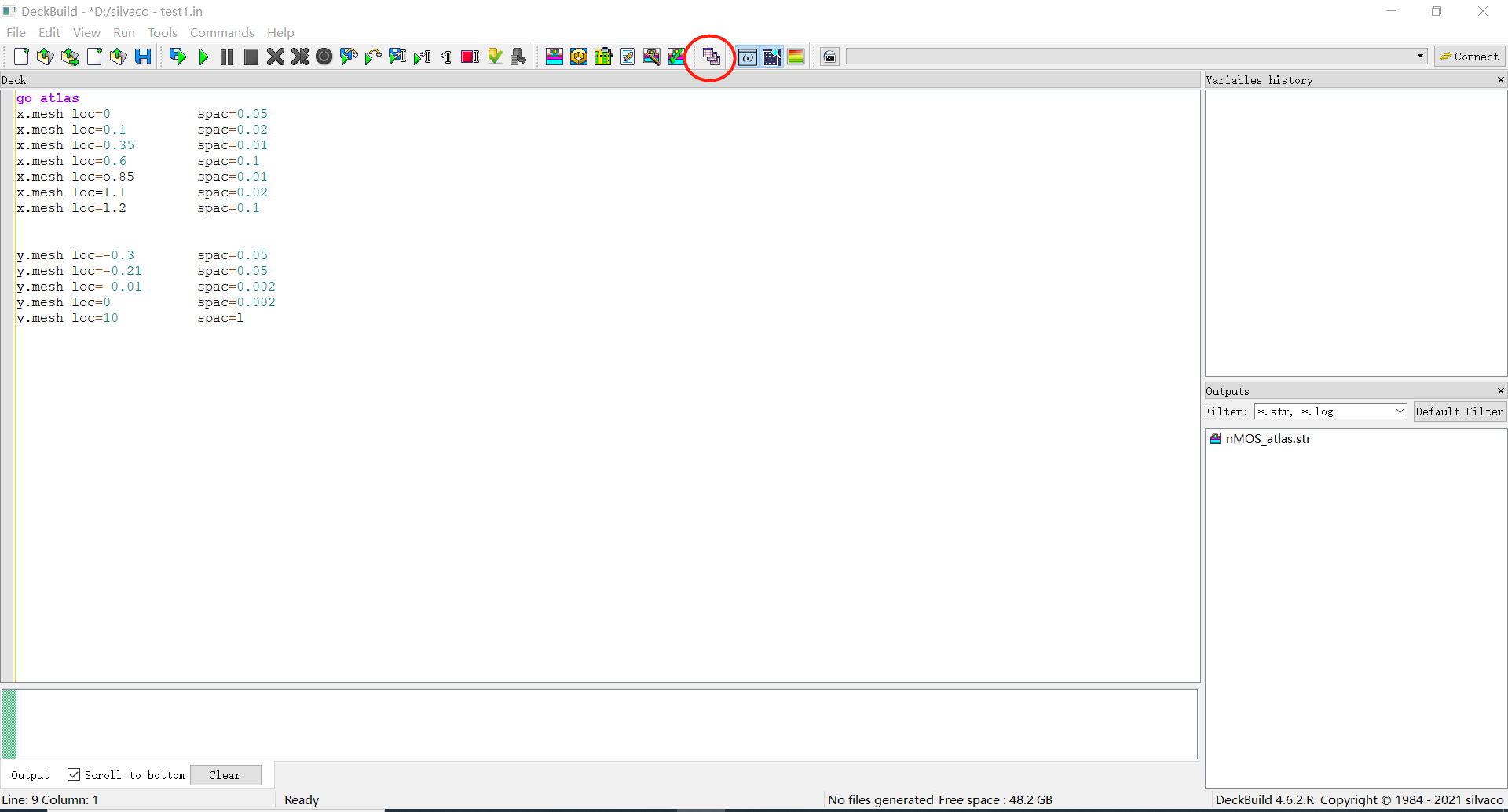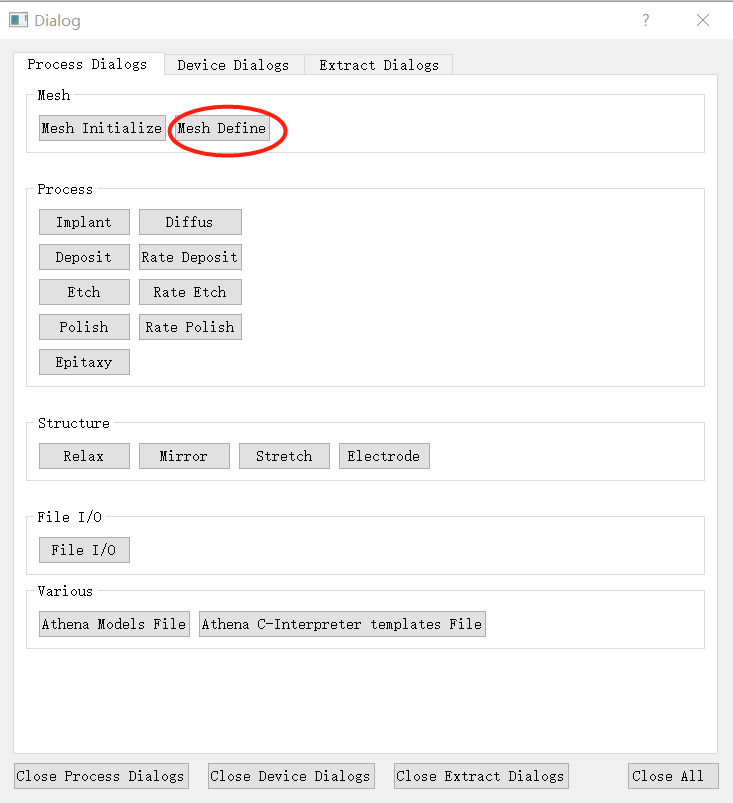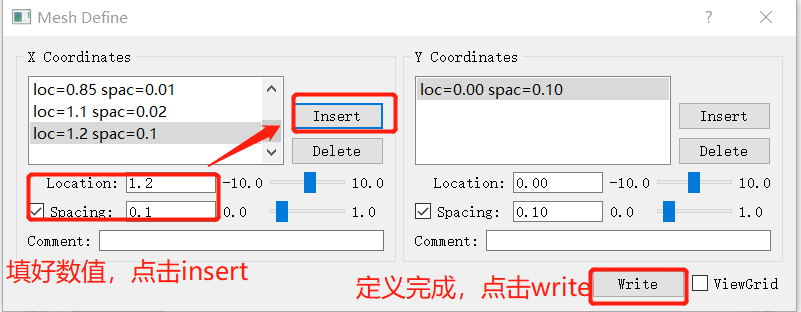网格计算：

实际的物理系统非常复杂，连续系统的信息量也巨大到无法估量，必须将其离散化。所以应用网格计算进行半导体仿真应用。

网格计算，将半导体仿真区域划分成网格，在网格点处计算出希望得到的特性（如：电学性质，光学性质，工艺步骤的速率等等）。

精细的网格能得到较精确的结果，但相应地会增加计算时间，也可能导致不收敛。

• 由网格线以及网格线之间的间隙来 描述仿真区域的网格；
• 通过网格释放来使后续步骤中不是很紧要的区域的网格点变少， 网格释放之后也可以再重新建立合适的精细的网格。
• 用三角形参数来控制网格的长宽比。 如果将矩形网格的对角线相连，则可以形成两个三角形，控制三角形的角度就可控制网格的 长宽比；
• 在适当的区域增删网格线。

### Structure Specification ——region、electrode

• mesh
• region
• electrode
• doping

``````region number=1  x.min=0     x.max=1.2   y.min=0     y.max=10    material=silicon
region number=2  x.min=0     x.max=1.2   y.min=-0.3  y.max=0     material=oxide
region number=3  x.min=0.35  x.max=0.85  y.min=-0.21 y.max=-0.01 material=poly
region number=4  x.min=0.35  x.max=0.85  y.min=-0.3  y.max=-0.21 material=aluminum
region number=5  x.min=0     x.max=0.1   y.min=-0.3  y.max=0.0   material=aluminum
region number=6  x.min=1.1   x.max=1.2   y.min=-0.3  y.max=0.0   material=aluminum

electrode reg=4  name=gate
electrode reg=5  name=source
electrode reg=6  name=drain
electrode bottom name=substrate
``````

区域划分成region1、region2、region3.。。。

region的大小由x.min、 x.max、 y.min 、y.max 决定。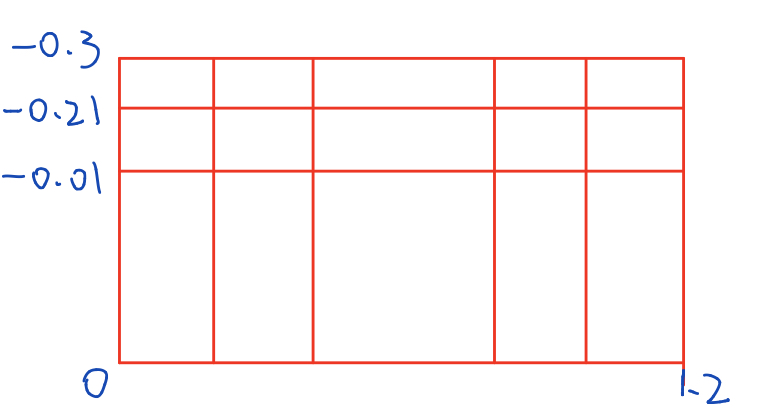确定每个region的材料，电极以region的方式给。（填充的时候，时候后面region压在前面region上）（怎么理解：当作乐高积木拼上去）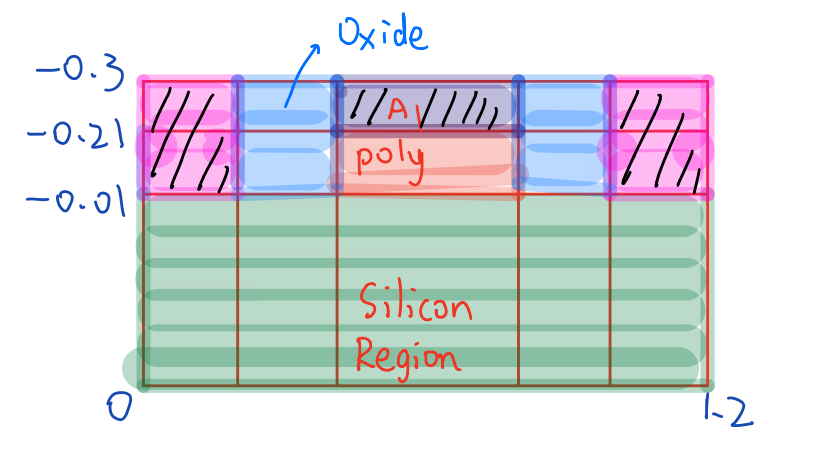### Structure Specification ——doping

• mesh
• region
• electrode
• doping

掺杂：

``````//放在哪个区域里面进行掺杂
//region1（Si的位置）是p.type均匀掺杂，掺杂浓度是1e17
//region3（poly的位置）是p.type均匀掺杂，掺杂浓度是5e20
doping uniform region=1   x.min=0     x.max=1.2   y.min=0     y.max=10    p.type concentration=1e17
doping uniform region=3   x.min=0.35  x.max=0.35  y.min=-0.21 y.max=-0.01 n.type concentration=5e20
doping gauss   region=1   x.min=0     x.max=1.2   junc=0.02   rat=0.6     p.type concentration=5e17
doping gauss   region=1   x.min=0     x.max=0.35  junc=0.05   rat=0.6     n.type concentration=4e10
doping gauss   region=1   x.min=0.85  x.max=1.2   junc=0.05   rat=0.6     n.type concentration=4e18
doping gauss   region=1   x.min=0     x.max=0.15  junc=0.2    rat=0.6     n.type concentration=5e20
doping gauss   region=1   x.min=1.05  x.max=1.2   junc=0.2    rat=0.6     n.type concentration=5e20

//uniform意思是采用均匀掺杂
//gauss，高斯分布，非均匀掺杂，比较趋近foundry做出的样子``````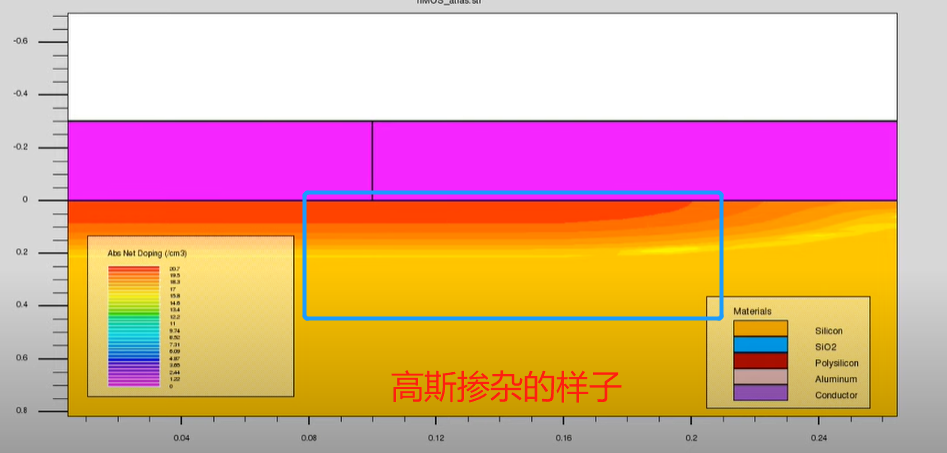save outf=ooxx1.str

（注意每次save要“分段”save，一次跑一行，慢慢存，不可一次写完，从头跑到尾巴、，程序会卡住）

### Model Specification ——models

• models
• contact
• interface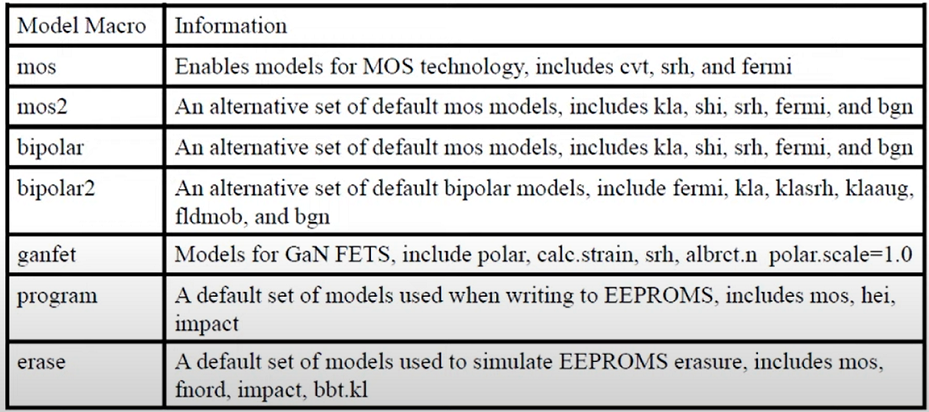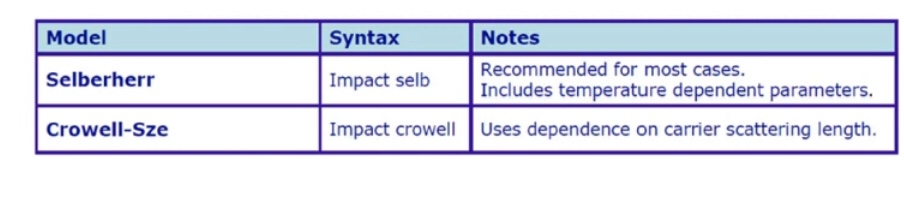mos结构常用第一个模型。

### Model Specification ——contact

• models
• contact
• interface

Atlas supports several boundary conditions：

• Ohmic contacts（默认情况下是ohmic contact 。）
• Schottky contacts
• Current boundary conditions
• Lumped elements between applied biases and device contacts
• Distributed contact resistance to take into account the finite resistivity of semiconductor contacts.

Ohmic Contacts

``CONTACT NAME=ANODE NEUTRAL``

Schottky Contacts

要设置肖特基接触，请使用 CONTACT 语句的 WORKFUN 参数指定功函数 (eV)：

``CONTACT NAME=BODY WORKFUN=4.94``

在实际中，功函数定义为

【 AFFINITY是亲和力，  是金属-半导体界面处的势垒高度，单位为 eV。】

例如，

如果肖特基接触是AI，与Si的功函数差为 4.2eV，势垒高度为 0.7eV，则 WORKFUN=4.9

Current boundary conditions

current boundary conditions计算由 CONTACT 语句中的 CURRENT 参数激活：

``CONTACT NAME=BASE CURRENT``

当电流对电压高度敏感或者是电压的多值函数时，电流控制电极很有用

（post-breakdown and snap-back） 。

External Resistor,Capacitors, Inductors

可以使用 CONTACT 语句中的 RESISTANCE、CAPACITANCE 和 INDUCTANCE 参数指定连接到电极的电阻、电容和电感：

``CONTACT NAME=DRAIN CAPACITANCE=20e-12``

由于接触材料具有有限的电阻率，沿金属半导体表面的静电势并不总是均匀的。为了解决这种影响，可以使用 CON.RESIST 参数将分布接触电阻与任何电极相关联：

``CONTACT NAME=SOURCE CON.RESITANCE=0.01``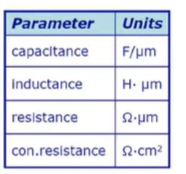Floating Contacts

``CONTACT NAME=SOURCE RESISTANCE=1e14``

### Model Specification ——contact

• models
• contact
• interface

INTERFACE 语句指定半导体绝缘体边界处的界面参数。 除非另有说明，所有参数仅适用于边界节点。

``````INTERFACE [<params>]
``````

INTERFACE 语句由一组界面的边界条件参数和一组用于定位这些参数effect的参数组成  。

``````INTERFACE qf=3e10
``````

该语句指定半导体和绝缘体之间的所有界面都具有 3e10 cm-2 的固定电荷。

可以使用 x.min，x.max，y.min 和 y.max 将感兴趣的区域限制在特定区域  。

``````INTERFACE x.min=-4 x.max=4 y.min=-e.5 y.max=4 qf=1e10 s.n=1e4 s.p=1e4
``````

该语句定义了具有固定电荷和复合速度的接口

### Numerical Method Selection ——method

• method

``````method newton itlimit=25 maxtrap=4
``````
• newton：使用牛顿法获得非线性解。 ltlimit：指定允许的最大迭代次数（default=25）
• maxtrap：指定在发散的情况下陷阱过程将重复的次数（default=4）。 maxtrap=10 推荐用于击穿模拟。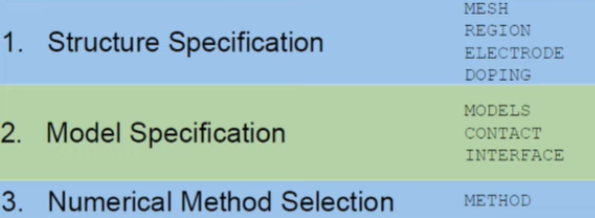``````go atlas simflags="-P 2"
mesh inf=nMOs_atlas.str //之前第一步定义的结构

models mos    //定义model
interface qf=3e10  //interface

method newton itlimit=25 maxtrap=4  //method
``````

### Solution Specification ——log

• log
• solve
• save
``````solve init   //几个电极归零解，解一次。
solve ....#     //要解的内容

log outfile=xxx.log  //要解的数值放在哪里，xxx是自己定义的名字
solve .....##
log off

save outfile=xxx.str //存档
``````
``````//上面...#里面的东西，是要解的内容：
//nmos可以解什么：
IdVg
Idvd
BVoff

//量取的方法（后面是量测条件）：
a. IdVg @ Vd=0.1, Vg=0 ~3V,Vs=0,Vb=0
//给Vd 0.1，Vg扫0-3V，然后Vs接0，Vb接0

b. Idvd @ Vg=1,2,3V,Vd=0 ~ 5V,Vs=0,Vb=0
c. BVoff @ vd= 0 sweep I=1-6A

``````

### Result Analysis

• extract
• tonyplot

萃取Vthreshold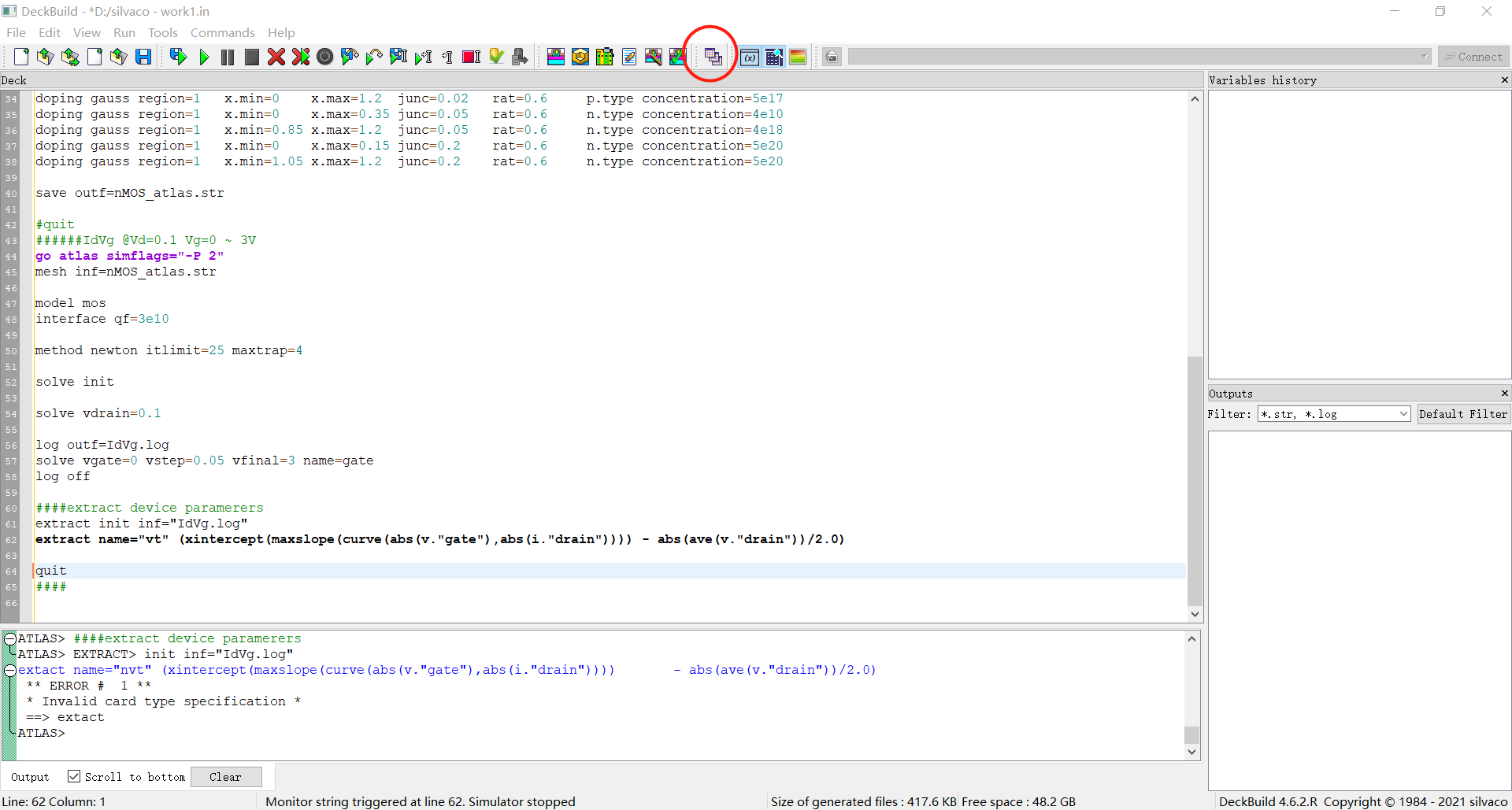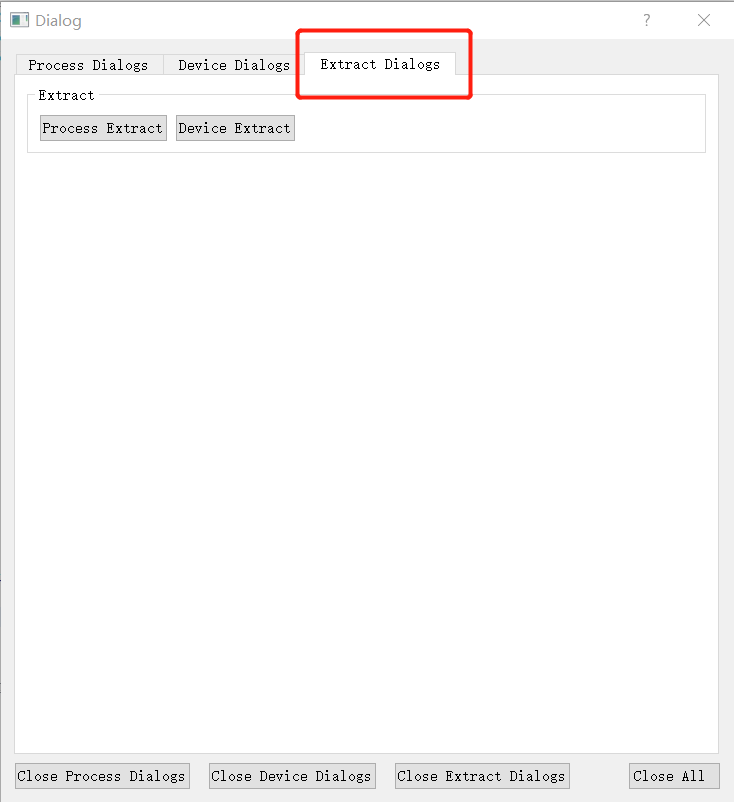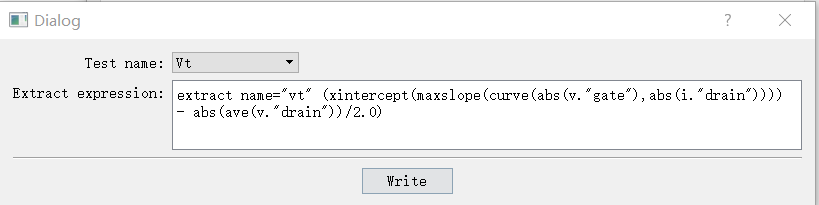点击write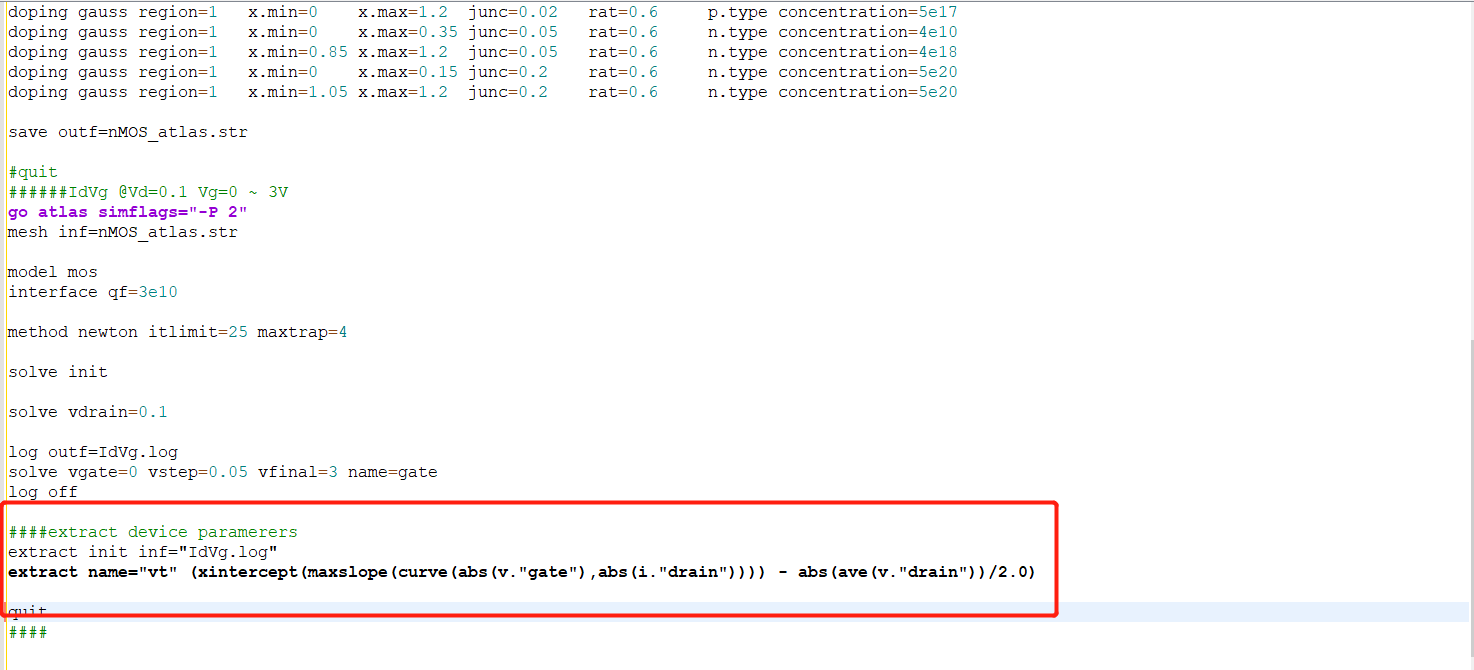### Result Analysis

• extract
• tonyplot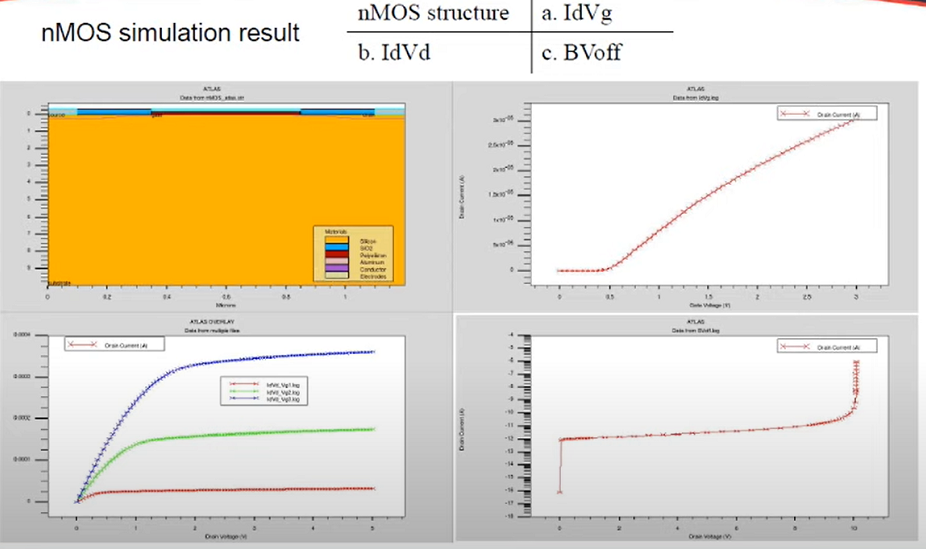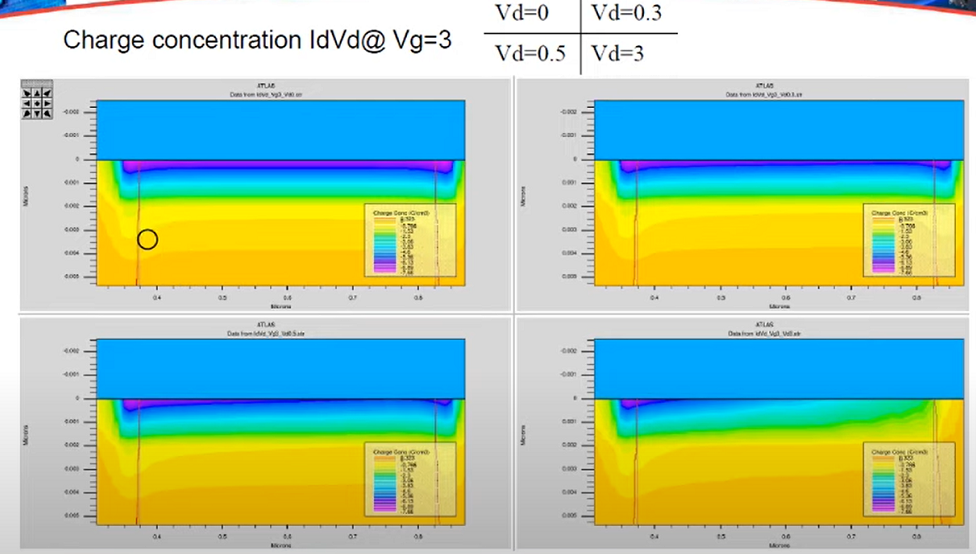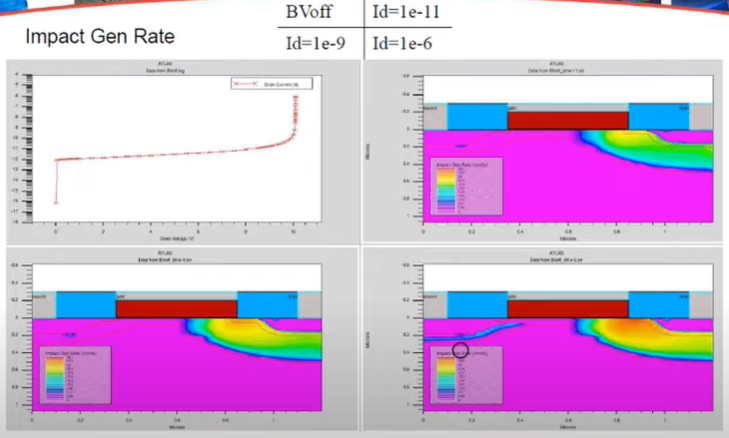a. IdVg @ Vd=0.1, Vg=0 ~3V,Vs=0,Vb=0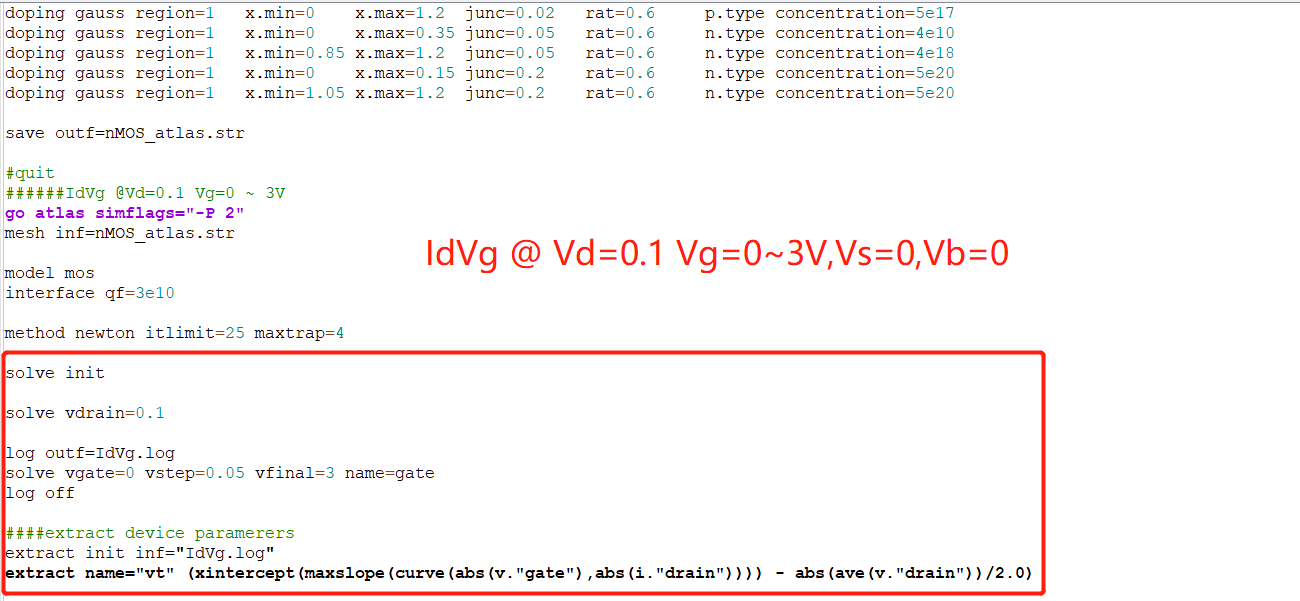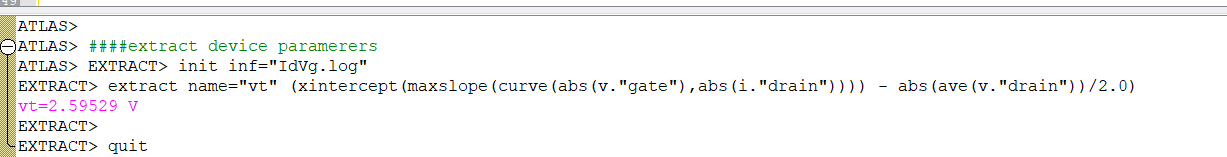IdVd @Vg=1,2,3 Vd=0 ~ 5V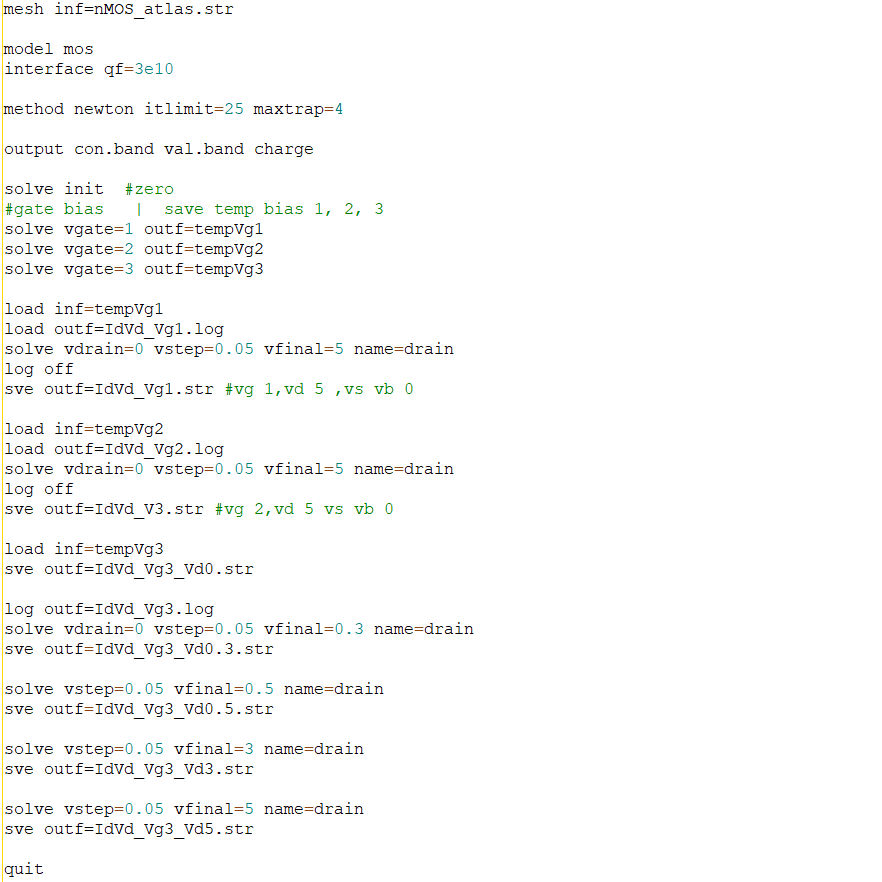参考：

Silvaco学习日记（2020/10/20）